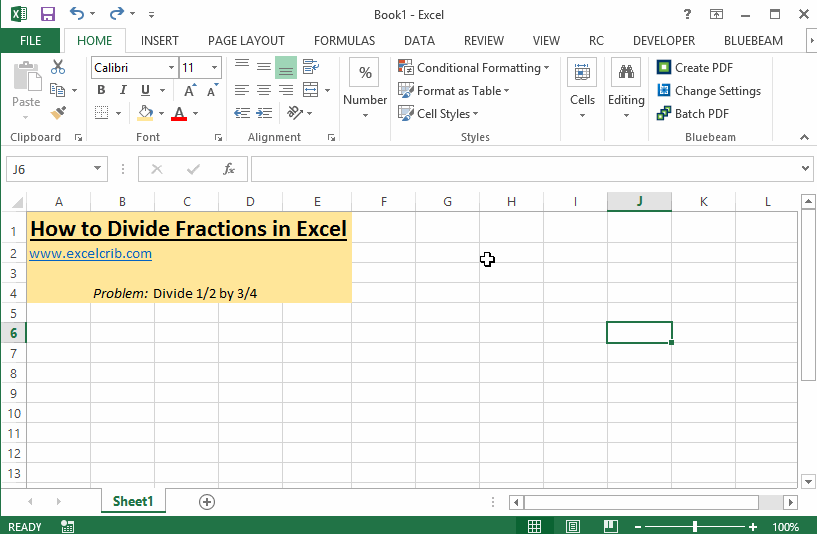# How to Divide Fractions in Excel

Microsoft Excel are capable of solving fraction problems. This tutorial will show how to divide fractions.

## Steps

1. Input the fractions as a regular formula in individual cells. Excel will automatically convert it to decimal format. For example =1/2 will yield 0.5 in a cell.
2. Divide the numbers using cell reference separated by / symbol. Again Excel will convert it into a decimal formal.
3. Select the cells where you want to convert to fraction format, then format cells (can be accessed by either right-clicking or from the ribbon menu). A small window will pop out, then select 'Number' tab, choose 'Fraction' and press 'OK'.

Watch the screen recording below for demonstration.You might also want to learn how to multiply fractions, see our previous tutorial.